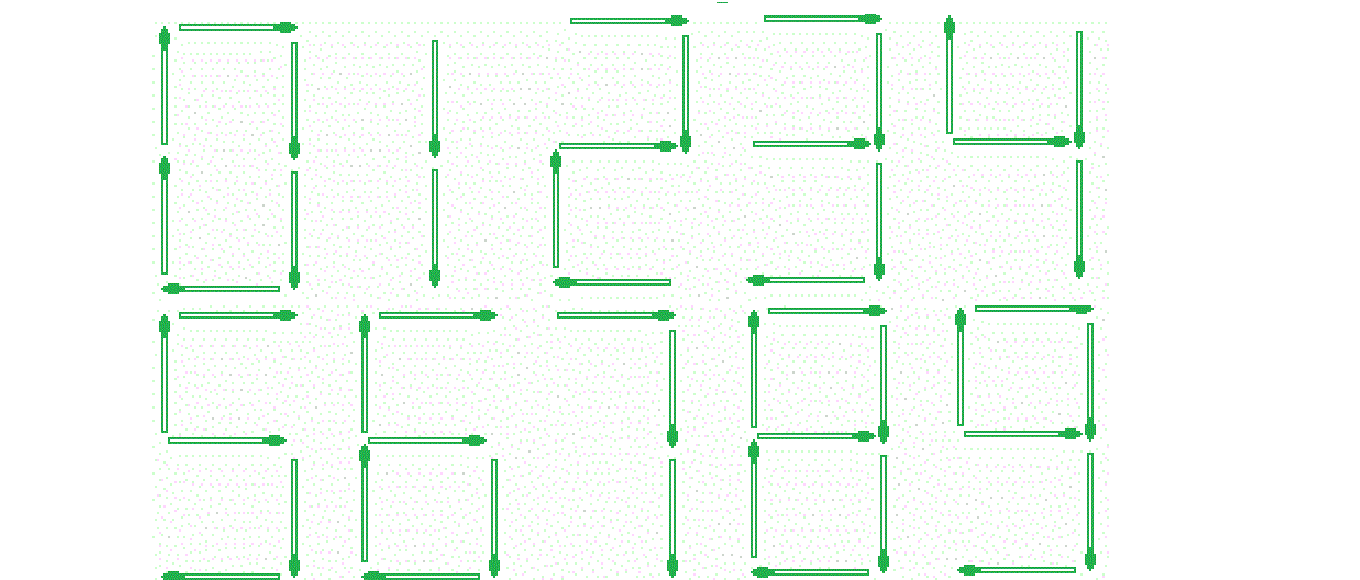# Count of matchsticks required to represent the given number

Given a large integer as a string str, the task is find the number of matchsticks required to represent it.Examples:

Input: str = “56”
Output: 11
5 sticks are required to represent 5 and
6 sticks are required to represent 6.

Input: str = “548712458645878”
Output: 74

## Recommended: Please try your approach on {IDE} first, before moving on to the solution.

Approach: Store the count of match sticks required to represent every digit from 0 to 9 in an array sticks[]. Now traverse the given string digit by digit and add the count of sticks required for the current digit.

Below is the implementation of the above approach:

## C++

 `// C++ implementation of the approach ` `#include ` `using` `namespace` `std; ` ` `  `// stick[i] stores the count of sticks ` `// required to represent the digit i ` `const` `int` `sticks[] = { 6, 2, 5, 5, 4, 5, ` `                       ``6, 3, 7, 6 }; ` ` `  `// Function to return the count of ` `// matchsticks required to represent ` `// the given number ` `int` `countSticks(string str, ``int` `n) ` `{ ` `    ``int` `cnt = 0; ` ` `  `    ``// For every digit of the given number ` `    ``for` `(``int` `i = 0; i < n; i++) { ` ` `  `        ``// Add the count of sticks required ` `        ``// to represent the current digit ` `        ``cnt += (sticks[str[i] - ``'0'``]); ` `    ``} ` ` `  `    ``return` `cnt; ` `} ` ` `  `// Driver code ` `int` `main() ` `{ ` `    ``string str = ``"56"``; ` `    ``int` `n = str.length(); ` ` `  `    ``cout << countSticks(str, n); ` ` `  `    ``return` `0; ` `} `

## Java

 `// Java implementation of the approach ` `import` `java.util.*; ` ` `  `class` `GFG  ` `{ ` ` `  `// stick[i] stores the count of sticks ` `// required to represent the digit i ` `static` `int` `sticks[] = { ``6``, ``2``, ``5``, ``5``, ``4``, ``5``, ` `                        ``6``, ``3``, ``7``, ``6` `}; ` ` `  `// Function to return the count of ` `// matchsticks required to represent ` `// the given number ` `static` `int` `countSticks(String str, ``int` `n) ` `{ ` `    ``int` `cnt = ``0``; ` ` `  `    ``// For every digit of the given number ` `    ``for` `(``int` `i = ``0``; i < n; i++) ` `    ``{ ` ` `  `        ``// Add the count of sticks required ` `        ``// to represent the current digit ` `        ``cnt += (sticks[str.charAt(i) - ``'0'``]); ` `    ``} ` `    ``return` `cnt; ` `} ` ` `  `// Driver code ` `public` `static` `void` `main(String []args)  ` `{ ` `    ``String str = ``"56"``; ` `    ``int` `n = str.length(); ` ` `  `    ``System.out.println(countSticks(str, n)); ` `} ` `} ` ` `  `// This code is contributed by 29AjayKumar `

## Python3

 `# Python3 implementation of the approach  ` ` `  `# stick[i] stores the count of sticks  ` `# required to represent the digit i  ` `sticks ``=` `[ ``6``, ``2``, ``5``, ``5``, ``4``, ``5``,  ` `           ``6``, ``3``, ``7``, ``6` `];  ` ` `  `# Function to return the count of  ` `# matchsticks required to represent  ` `# the given number  ` `def` `countSticks(string, n) : ` ` `  `    ``cnt ``=` `0``;  ` ` `  `    ``# For every digit of the given number  ` `    ``for` `i ``in` `range``(n) : ` ` `  `        ``# Add the count of sticks required  ` `        ``# to represent the current digit  ` `        ``cnt ``+``=` `(sticks[``ord``(string[i]) ``-` `ord``(``'0'``)]);  ` ` `  `    ``return` `cnt;  ` ` `  `# Driver code  ` `if` `__name__ ``=``=` `"__main__"` `:  ` ` `  `    ``string ``=` `"56"``;  ` `    ``n ``=` `len``(string);  ` ` `  `    ``print``(countSticks(string, n));  ` ` `  `# This code is contributed by AnkitRai01 `

## C#

 `// C# implementation of the approach ` `using` `System; ` `                     `  `class` `GFG  ` `{ ` ` `  `// stick[i] stores the count of sticks ` `// required to represent the digit i ` `static` `int` `[]sticks = { 6, 2, 5, 5, 4, 5, ` `                        ``6, 3, 7, 6 }; ` ` `  `// Function to return the count of ` `// matchsticks required to represent ` `// the given number ` `static` `int` `countSticks(String str, ``int` `n) ` `{ ` `    ``int` `cnt = 0; ` ` `  `    ``// For every digit of the given number ` `    ``for` `(``int` `i = 0; i < n; i++) ` `    ``{ ` ` `  `        ``// Add the count of sticks required ` `        ``// to represent the current digit ` `        ``cnt += (sticks[str[i] - ``'0'``]); ` `    ``} ` `    ``return` `cnt; ` `} ` ` `  `// Driver code ` `public` `static` `void` `Main(String []args)  ` `{ ` `    ``String str = ``"56"``; ` `    ``int` `n = str.Length; ` ` `  `    ``Console.WriteLine(countSticks(str, n)); ` `} ` `} ` ` `  `// This code is contributed by 29AjayKumar `

Output:

```11
```

Time Complexity: O(n)

Attention reader! Don’t stop learning now. Get hold of all the important DSA concepts with the DSA Self Paced Course at a student-friendly price and become industry ready.

My Personal Notes arrow_drop_upCheck out this Author's contributed articles.

If you like GeeksforGeeks and would like to contribute, you can also write an article using contribute.geeksforgeeks.org or mail your article to contribute@geeksforgeeks.org. See your article appearing on the GeeksforGeeks main page and help other Geeks.

Please Improve this article if you find anything incorrect by clicking on the "Improve Article" button below.

Improved By : AnkitRai01, 29AjayKumar